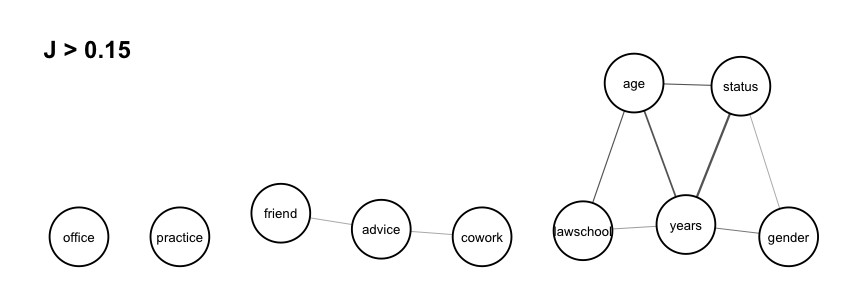# Joint Entropies and Association Graphs

In the section on univariate, bivariate and trivariate entropies, we saw that the bivariate entropy of two variables $$X$$ and $$Y$$ is bounded according to $H(X) \leq H(X,Y) \leq H(X)+H(Y) \ .$ The increment between the upper bound and the bivariate entropy is equal to the joint entropy given by $J(X,Y) = H(X)+H(Y)-H(X,Y)$ and is a non-negative measure of dependence or association between variable $$X$$ and $$Y$$. It is equal to 0 if and only if $$X$$ and $$Y$$ are stochastically independent $$X\bot Y$$ such that the probability of any bivariate outcome is the product of the probabilities of the univariate outcomes, i.e. $$p(x,y)=p(x,+)p(+,y)$$.

Trivariate entropies for triples of variables $$X,Y,Z$$ are bounded by $H(X,Y) \leq H(X,Y,Z) \leq H(X,Z) + H(Y,Z) - H(Z)$ The increment between the upper bound and the trivariate entropy is equal to the expected joint entropy given by $EJ(X,Y|Z) = H(X,Z)-H(Y,Z)-H(Z)-H(X,Y,Z)$ which is non-negative and equal to 0 if and only if we have conditional independence of the form $$X\bot Y | Z$$. Thus, it can be used to measure the deviation from conditional independence.

The joint entropies $$J(X,Y)$$ for all pairs of variables $$X$$ and $$Y$$ are used to check dependence structure by looking at a sequence of association graphs constructed with nodes representing the variables and links between any pair of nodes corresponding to two variables with joint entropy above a chosen threshold. By successively lowering the threshold from the maximum joint entropy to smaller occurring values, the sequence of graphs get more and more links. Connected components that are cliques represent dependent subsets of variables, and different components represent independent subsets of variables. Conditional independence between subsets of variables can be identified by omitting the subset corresponding to the conditioning variables. A threshold that gives a graph with reasonably many small independent or conditionally independent subsets of variables can be considered to represent a multivariate model for further testing.

## Example: joint entropies and association graphs

library(netropy)

We create a dataframe dyad.var consisting of dyad variables as described and created in variable domains and data editing. Similar analyses can be performed on observed and/or transformed dataframes with vertex or triad variables.

head(dyad.var)
##   status gender office years age practice lawschool cowork advice friend
## 1      3      3      0     8   8        1         0      0      3      2
## 2      3      3      3     5   8        3         0      0      0      0
## 3      3      3      3     5   8        2         0      0      1      0
## 4      3      3      0     8   8        1         6      0      1      2
## 5      3      3      0     8   8        0         6      0      1      1
## 6      3      3      1     7   8        1         6      0      1      1

The function joint_entropy() computes the joint entropies between all pairs of variables in a given dataframe and returns a list consisting of the upper triangular joint entropy matrix (univariate entropies in the diagonal) and a dataframe giving the frequency distributions of unique joint entropy values. A function argument specifies the precision given in number of decimals for which the frequency distribution of unique entropy values is created (default is 3). Applying the function on the dataframe dyad.var with two decimals:

J <- joint_entropy(dyad.var, 2)
J$matrix ## status gender office years age practice lawschool cowork advice ## status 1.49 0.17 0.09 0.79 0.38 0.00 0.08 0.02 0.05 ## gender NA 1.55 0.03 0.28 0.07 0.00 0.06 0.00 0.01 ## office NA NA 2.24 0.08 0.14 0.05 0.13 0.06 0.10 ## years NA NA NA 2.67 0.61 0.05 0.20 0.02 0.05 ## age NA NA NA NA 2.80 0.02 0.41 0.01 0.02 ## practice NA NA NA NA NA 1.96 0.04 0.05 0.08 ## lawschool NA NA NA NA NA NA 2.95 0.00 0.01 ## cowork NA NA NA NA NA NA NA 0.62 0.18 ## advice NA NA NA NA NA NA NA NA 1.25 ## friend NA NA NA NA NA NA NA NA NA ## friend ## status 0.05 ## gender 0.01 ## office 0.08 ## years 0.07 ## age 0.05 ## practice 0.01 ## lawschool 0.02 ## cowork 0.04 ## advice 0.18 ## friend 0.88 J$freq
##       j  #(J = j) #(J >= j)
## 1  0.79         1         1
## 2  0.61         1         2
## 3  0.41         1         3
## 4  0.38         1         4
## 5  0.28         1         5
## 6   0.2         1         6
## 7  0.18         2         8
## 8  0.17         1         9
## 9  0.14         1        10
## 10 0.13         1        11
## 11  0.1         1        12
## 12 0.09         1        13
## 13 0.08         4        17
## 14 0.07         2        19
## 15 0.06         2        21
## 16 0.05         7        28
## 17 0.04         2        30
## 18 0.03         1        31
## 19 0.02         5        36
## 20 0.01         5        41
## 21    0         4        45

As seen, the strongest association is between the variables status and years with joint entropy values of 0.79. We have independence (joint entropy value of 0) between two pairs of variables: (status,practice), (practise,gender), (cowork,gender),and (cowork,lawschool).

These results can be illustrated in a association graph using the function assoc_graph() which returns a ggraph object in which nodes represent variables and links represent strength of association (thicker links indicate stronger dependence). To use the function we need to load the ggraph library and to determine a threshold which the graph drawn is based on. We set it to 0.15 so that we only visualize the strongest associations:

library(ggraph)
assoc_graph(dyad.var, 0.15)Given this threshold, we see isolated and disconnected nodes representing independent variables. We note strong dependence between the three dyadic variables status,years and age, but also a somewhat strong dependence among the three variables lawschool, years and age, and the three variables status, years and gender. The association graph can also be interpreted as a tendency for relations cowork and friend to be independent conditionally on relation advice, that is, any dependence between dyad variables cowork and friend is explained by advice.